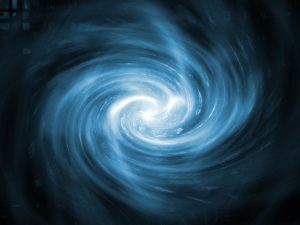In this article we will finish up our discussion of the toroidal nature of the Aether and the torus as the heart of the Aether unit.

We will take yet another perspective, entering into the discussion through the avenue of number, specifically the interesting ideas of Vortex Based Mathematics.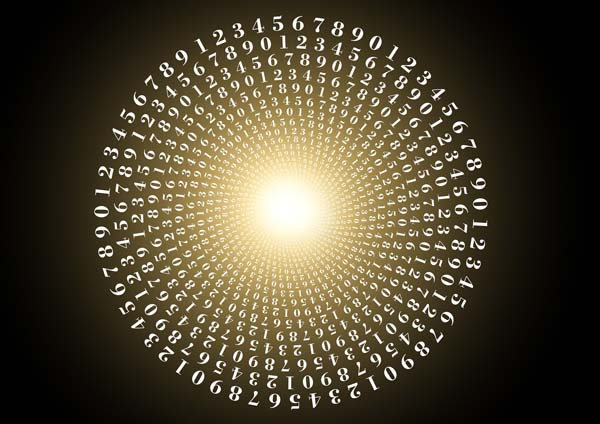“All is Number.”  Pythagoras

Vortex Based Mathematics (VBM)

Vortex Based Mathematics was discovered by Marko Rodin and developed further by Randy Powell.1

It is a dynamic math that shows the relationships and thus, qualities, of numbers rather than mere quantities.

Human beings did not invent the concept of quantities – we merely assigned a symbol to represent these quantities.

Numbers are stationary vector interstices that are real and exhibiting at all times spatial, temporal, and volumetric qualities.

Being stationary means they are fixed constants.

Vortex Based Mathematics was founded on base-10 math and the “omni-potent” number 9.

According to Rhuben Neal, writing about Vortex Based Mathematics, “Numbers are Real and Alive not merely symbols for other things.  You will discover that the relationships between numbers are not random or man-made but that numbers are actually elementary particles of which everything is composed.  This lost knowledge was well-known to our ancients and is now being uncovered for us today. Gradually you will come to see numbers in a simple yet profoundly perfect three-dimensional matrix grid pattern that forms the shape of a torus.

I would say it like this; everything is trinary in truth and binary in illusion, the decimal system has a left and right side (0.0) only as a result of the point in the centre which is the third “invisible” part that creates the “binary”. One side is macro, one side is micro. The point in the centre is where you are. The centre of radiant consciousness. The third point. The point of awareness that perceives space-time subjectively, dualistically, in order to have an “experience” of separation from the ALL.  Number systems are the closest thing we have outside of experience directly of raw energy, the prime power of the matrix construct. Since numbers can be used to understand and view geometry, sound, light and dynamics they are primary to all things in terms of a tool of creation.”2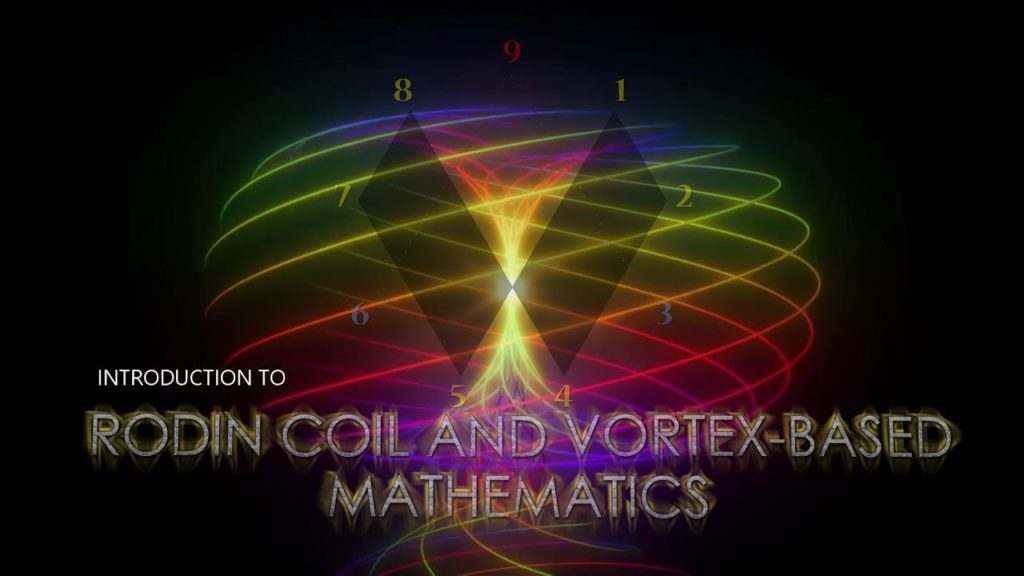Reducing a Number Down to One Digit

Reducing a number down to one digit is both popular in new age circles and numerology, and it is also a verifiable form of math commonplace in universities worldwide.

This process is called by many names:

integrated digits (Buckminster Fuller); indig 9; digital root, digital sum, quantum bit (computer science); Kabbalistic reduction; Pythagorean addition; decimal parity; quantum numerology; indig; number essence; reduced ordinal values; horizontal addition; numeric reduction

The process is extremely simple.  For example:

19        = 1 + 9 = 10 = 1 + 0 = 1

1546    = 1 + 5 + 4 + 6 = 16 = 1 + 6 = 7

333      = 3 + 3 + 3 = 9

And so on.

The “Mathematical Fingerprint of God”

The Mathematical Fingerprint of God in VBM is all based on the principle of doubling – 1, 2, 4, 8, 16, 32, 64, 128…etc.

Why doubling?  Because doubling represents the growth in octaves and all in reality grows in octaves.

As Walter Russell writes, “Waves are electric pressure-conditioned octaves of tones…Every element in the entire periodic table is a transmutation from the preceding element of its cycle, from its beginning in zero to the ending of the entire nine octaves in the zero of its beginning.”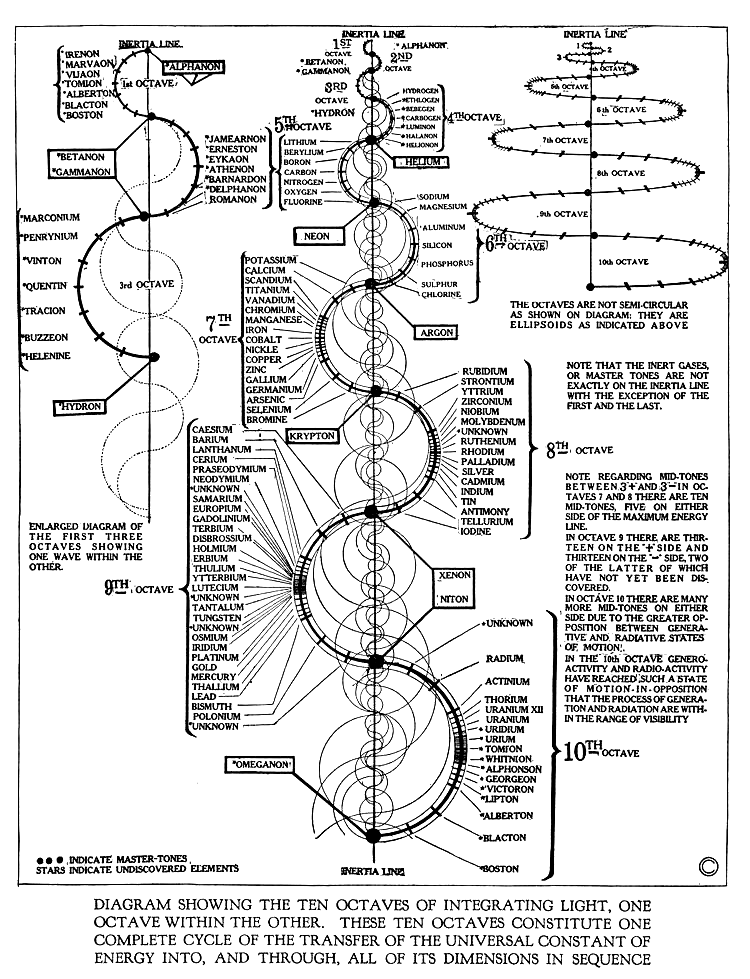Walter Russell’s Periodic Table of Elements as a spiral of nine octaves.  Credit: Walter Russell

To discover the Mathematical Fingerprint of God:

Draw a circle.

Divide the circumference of the circle into 9 equal parts (40 degrees each).

Put 9 at the top and 1 through 8 going clockwise around the circle.

Next Instructions: To Find the Sequence: 1-2-4-8-7-5-1…

Start at 1.

Move to the next “double” = 2

Next double = 4

Next double = 8

Next double = 16 (1+6=7)  Next number is 7

Next double = 32 (3+2=5)  Next number is 5

Next double = 64 (6+4=10 = 1+0=1)  Next number is 1.

You end where you begin.

A straight-line infinity sign forms from the sequence 1, 2, 4, 8, 7, 5, 1…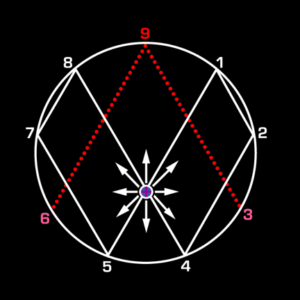The sequence will repeat in this fashion if you keep going with the doubling.

128 = 1+2+8=11 = 1+1 = 2

256 = 2+5+6=13 = 1+3 = 4

512 = 5+1+2 = 8

1024 = 1+2+4 = 7

2048 = 2+4+8=14 = 1+4 = 5…etc.

The number sequence stays intact as you half numbers – it just goes in the opposite direction.

Start at 1.

Go back half = .5 = 5

Next half = .25 = 2 + 5 = 7

Next half = .125 = 1+2+5 = 8

Next half = .0625 = 6+2+5 = 13 = 1+3 = 4

Next half = .03125 = 3+1+2+5 = 11 = 1+1 = 2

The 3, 6, 9

The 1, 2, 4, 8, 7, 5 sequence represents the physical reality.

3, 6, 9 represents the metaphysical reality – the Source reality.

There is a continual oscillation between 3 and 6.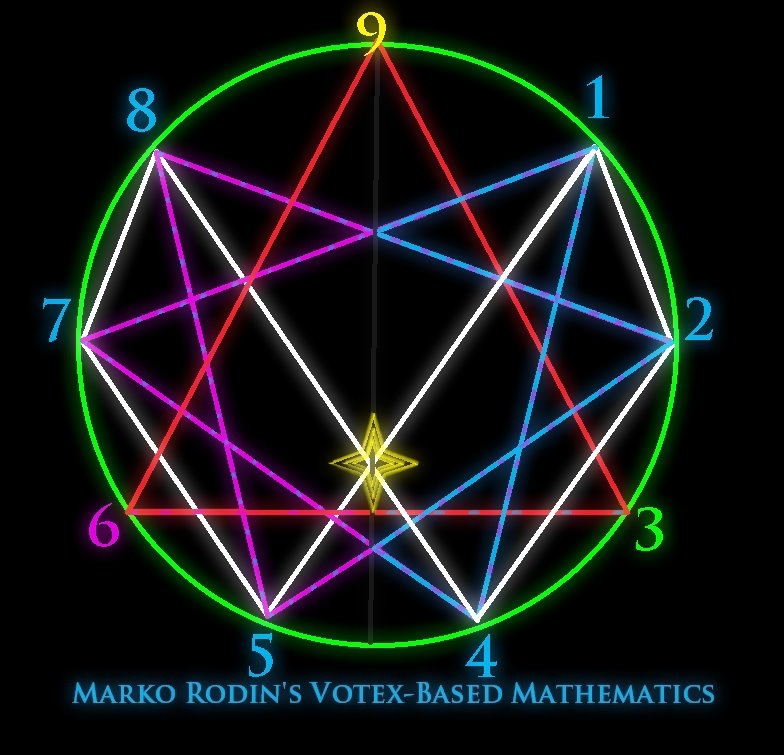3 doubled = 6

6 doubled = 12 = 1+2 = 3

12 doubled = 24 = 2+4 = 6

24 doubled = 48 = 4+8 = 12 = 1+2 = 3

48 doubled = 96 = 9+6 = 15 = 1+5 = 6

A pattern of 3,9,6,6,9,3,3,9 is created.

The 9

The 9 acts as a control – it is the “S” curve between the yin/yang with 3 and 6 representing the opposite sides.

9 represents energy being manifested in a single moment event of occurrence in our physical world of creation.

It is the focal center – the only number associated with an upright vertical axis.

It is the singularity point around which the octave revolves.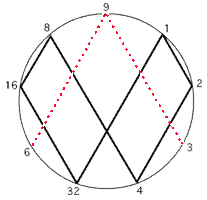9 lines up with the center of the Infinity sign.

The number 9 never changes.

All multiples of 9 equal 9.

9×1 = 9

9×2 = 18 = 1+8 = 9

9×3 = 27 = 2+7 = 9

9×4 = 36 = 3+6 = 9…etc.

The 9 is the center of the torus – the singularity point between the physical and metaphysical (space/time and time/space).

The metaphysical energy of the Aether/Consciousness/Spirit emanate from the center of mass outwards.

This creates a flow of the Aether.  This flow is the source of all movement and the source of the non-decaying spin of the electron.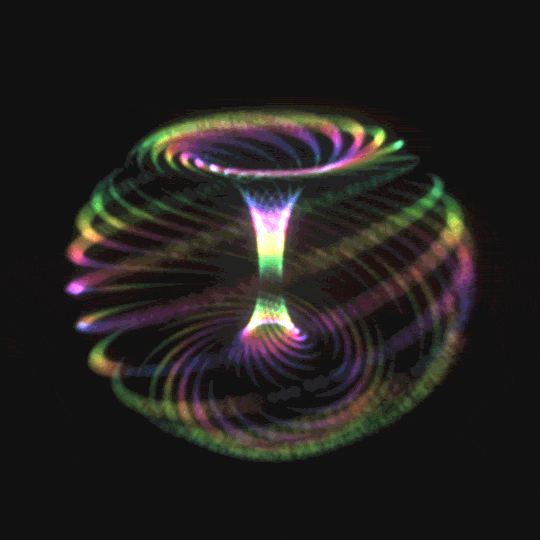Vortex Based Mathematics & the Aether3

In VBM Aether units are called “Aetherons” or “Aetheron Flux Monopole Emanations”.

Aetherons are the Life Force of the Universe.

Other names of the Aether: Spirit; Orgone; Prana; Essence of God; Fifth Force, Light of Eternity; Living Waters, Cosmic Rays.

They are responsible for all form and movement.

They are the source of all magnetic fields and create instantly reacting, high inductance, dual magnetic field flows.

They generate synchronized electricity.

These Aetheron emanations are the reason the torus exists.

They create nested vortices of energy flow.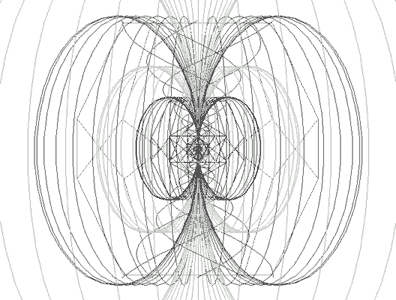The center of mass (of an atom) is devoid of mass; it is empty space.  There is aetheron emanation from the center of atomic nuclei.  The emanations impart non-decaying spin to electrons.  They determine angular properties of molecular and physical structure.  They are of positive polarity.

They create a negative draft counterspace, which is Gravity.

Aetherons are linear emanations of energy which originate from the center of all mass.

These emanations trigger cell regeneration.

Aether flow is the source of all movement.

All mass wraps around the Aetherons.  All movement curves around the aetheron flow.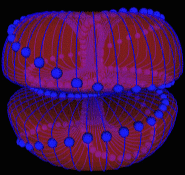Aether flow and the phenomenon of negative draft counterspace creates nested vortices on the torus.

Because of the aetheron flow, these vortices are present in the gap spaces between bound matter.

Polar Number Pairs

Numbers have bilateral (mirror) symmetry.

They line up in stratified layers of horizontal planes.

In VBM there are nine simple series – 1 for each number 1 through 9.

The series are based upon reducing all products to single digits via horizontal addition.

There are three polar number pairs:

1 and 8

2 and 7

• Multiples of 2:
• 1×2 = 2
• 2×2 = 4
• 3×2 = 6
• 4×2 = 8
• 5×2 = 10 = 1
• 6×2 = 12 = 3
• 7×2 = 14 = 5
• 8×2 = 16 = 7
• 9×2 = 18 = 9

4 and 5

• Multiples of 4:
• 1×4 = 4
• 2×4 = 8
• 3×4 = 12 = 3
• 4×4 = 16 = 7
• 5×4 = 20 = 2
• 6×4 = 24 = 6
• 7×4 = 28 = 1
• 8×4 = 32 = 5
• 9×4 = 36 = 9

Polar Pairs Series:

• [1,8] = 1,2,3,4,5,6,7,8 and 8,7,6,5,4,3,2,1
• [2,7] = 2,4,6,8,1,3,5,7 and 7,5,3,1,8,6,4,2
• [4,5] = 4,8,3,7,2,6,1,5 and 5,1,6,2,7,3,8,4

Everything starts from the center outwards.

The symmetry is a principle of nature.

The 9 axis causes the doubling circuit and it is the point towards which matter converges and away from which it diverges or expands.

The polar number pairs will be mirror images of each other, both flowing in opposite directions from the central axis.

There is perfect symmetry wrapped around a single point coiling outwards the way that petals are wrapped in a rose, or a nautilus shell spirals outward.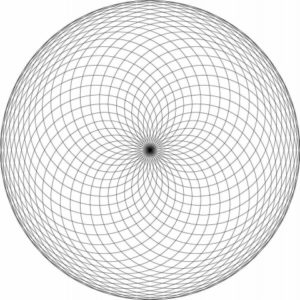Physics and Torus Structure

The base-10 decimal system is not man-made – it is created by this flow of energy discussed above.

It shows the dimensional shape and function of the universe as being a torus.

It uncovers the secret of bilateral symmetry as being the result of a diagonal motion along the surface and through the internal volume of the torus in an expanding and contracting polarized logarithmic spiral pattern produced by the flow of Aether – outward flow of EM energy; inward flow of gravity.

It reveals the pathway that all accelerated motion (gravity) seeks – spiral flow inwards.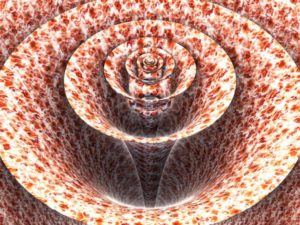It explains the workings of black holes and white holes – they are all various manifestations of the principles of compression and decompression.

The helical structure of DNA is also an example of this torsion effect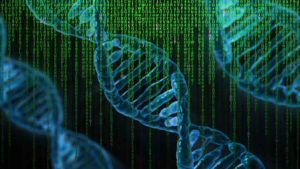The torus mapped here is a representation of all one-way living, systemic magnetic/electrical systems that suck things in at the top (implosion) and shoot them out at the bottom (explosion).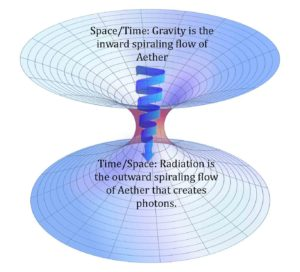Remember, Vector Based Mathematics Aether Units are called Aetherons.

Aetherons are omni-directional and emanate from the focal center of any body of mass outwards in a trinary harmonic phasing which is the source of the non-decaying spin of the electron and of inertia.

Aetherons penetrate everything and move in an undeviating, non-decaying straight line that all physical mass is forced to warp and curve around.

Aetherons bestow upon every material thing both its form and function.

They allow for the creation of ‘underpinning nested vortices’ similar to sunspots or dimples on a golf ball that have their own harmonically sequenced activation stages out of which a tremendous amount of information may be extrapolated.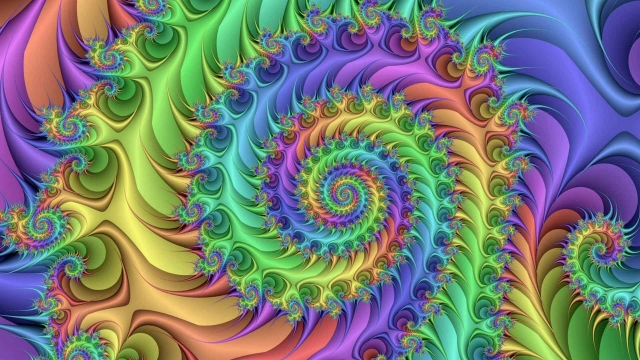The number map/torus produced is the only whole number numerical map in history that maintains a non-decaying scalar fractal pattern from micro infinity to macro infinite and yet is simultaneously a finite, contained, coherent, unified, and measurable entity.

It was also discovered that 1,2,4,8,7,5 was a doubling circuit for a very efficient electrical coil.

Conclusion

We have now taken a look at another viewpoint of the Aether and Aether units, this time through Vector Based Mathematics and Number.  There are many YouTube videos to watch on these topics to gain more understanding.

We have seen how Marko Rodin and Randy Powell have come to the same conclusions about Aether science as we have seen many others do.

This time, however, it is firmly based in the simple process of base-10 math, leading us back to where we started in Cosmic Core – that is, the study of number through the Pythagorean Quadrivium, reminding us that number is far more than mere quantities.  Number represents the cosmic creating archetypes of the universe that all in reality is based upon.